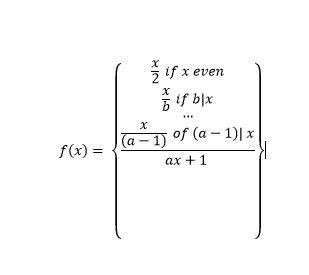## Recommended Posts

I think the approach is brilliant and maybe simple enough to work. But I am still not convinced.

You take 2 to a power and multiply by Prime factors. I agree that you can build any number this way, but I don’t believe multiplying by 2 here is the same as dividing an even number by 2 in the conjecture. The order of operations.

And I cannot test this algorithm in a computer since we cannot factor the numbers in this series.

Again I’m probably wrong but this is not a linear series. My understanding is that you are building a pattern linearly and the conjecture is not a one-to-one function.

This is just my understanding but if you can prove your method does this I will buy multiple copies when you get it published.

##### Share on other sites

1 hour ago, Trurl said:

I think the approach is brilliant and maybe simple enough to work. But I am still not convinced.

You take 2 to a power and multiply by Prime factors. I agree that you can build any number this way, but I don’t believe multiplying by 2 here is the same as dividing an even number by 2 in the conjecture. The order of operations.

And I cannot test this algorithm in a computer since we cannot factor the numbers in this series.

Again I’m probably wrong but this is not a linear series. My understanding is that you are building a pattern linearly and the conjecture is not a one-to-one function.

This is just my understanding but if you can prove your method does this I will buy multiple copies when you get it published.

Where do you think i'm multiplying by a power of two?   I'm doing nothing of the sort, I'm representing any natural number as a product of primes then to a sum of a prime.  The iterative approach in conjunction with the inequality property of a prime plus 1 , is how I create a power of 2 number.

take the number $$15$$ and the number $$18$$ then
for $$15$$:
$15 = 2^0 * 3*5 = \sum_{1}^{1*3}{5} = 5+5+5$
let $$w = 1*3$$
then
$\sum_{1}^{1*3 }{5} + w = (1 + 1 + 1) +5+5+5 = (5+1) + (5+1) + (5+1)$
Note:  each $$(5+1) < 2*5$$  therefore the all primes in $$5+1$$ are less than $$5$$

Notice the $$w$$ is the result of the iterative approach to adding 1 to each prime, and is allowed via the conjectures wording.  "For any natural number  $$n$$ there exists an $$i$$ such that $$f^i (n) = 1$$"  .  basically there is an $$i$$th iteration of the piece wise defined collatz function such that  $$f^i( n) = 1$$

$$18$$is solved similarly.
for $$18$$:
$18 = 2^1 * 3*3 = \sum_{1}^{2^1 * 3}{3} = 3+3+3+ 3+3+3$
let $$w = 2*3$$
then
$\sum_{1}^{2^1 * 3}{3} = 3+3+3+ 3+3+3 + w = (1 + 1 + 1 + 1 + 1 + 1 ) +3+3+3 +3+3+3= (3+1) + (3+1) + (3+1) + (3+1) + (3+1) + (3+1)$
clearly each $$(3+1) < 2*3$$ and again therefore all primes in $$(3+1)$$ are less than $$3$$

What happened to me was this:  While looking at the problem and breaking it into products of primes for each iteration, I began to wonder what the effect of adding 1 was.    I made an intuitive leap to looking at what happens when I add 1 to a single prime,  and therein lay the answer to the whole conjecture.   the behavior of "adding 1 to a single prime" is embedded in the problem.  The iterative nature implies we can add any number of 1's , which means we can "fully saturate" a sum of a prime. The multiplication by 3 in the collatz conjecture is irrelevant to the behavior, in fact we can look at a generalization and find a general formula to the collatz function.
for any prime $$a)\ the general collatz is as followsWhere \( b , c , ... ,(a-1)$$  are primes less than $$a$$  .  Note $$a-1$$ is not literally $$a -1$$,  it is the previous prime.
2 and 3 have a slightly special relationship as primes since they are right next to each other. So equations that do what the collatz does are simple to write,  primes further away result in a less simply defined "collatz" function.

Edited by Zolar V
##### Share on other sites

I think I have an understanding of what you are doing with factoring. You just want it in that form.

I would like to see an updated proof, since this thread has so many explanations.

This is the first time I saw this conjecture, so I hope some experts on this forum take interest.

The problem is worth working through. Right or wrong it is a fresh approach. Just because the conjecture is unsolved does not mean the idea isn’t valid.

##### Share on other sites

1 hour ago, Trurl said:

I think I have an understanding of what you are doing with factoring. You just want it in that form.

I would like to see an updated proof, since this thread has so many explanations.

This is the first time I saw this conjecture, so I hope some experts on this forum take interest.

The problem is worth working through. Right or wrong it is a fresh approach. Just because the conjecture is unsolved does not mean the idea isn’t valid.

I agree.  I really need to write the proof with the new information that has been garnered here.   That will have to wait since I am moving.   I really hope some experts take interest since I cannot find anything wrong with it.  The method seems right,  now I just have the daunting task of writing it well enough that other people can see what I see.   Thank you for your interest in the problem!

## Create an account

Register a new account